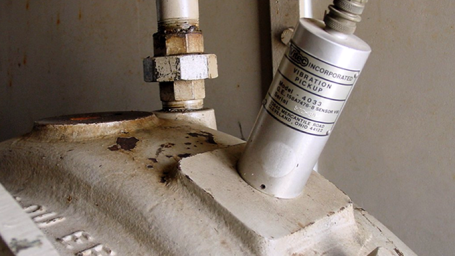Interactive

# Vibration Introduction

Martech
Updated Jan 21, 2021

After this lesson, you will be able to define basic terms and measurement units associated with vibration. You will also be able to describe the relationship between a machine’s operating speed and vibration problems. • Explain the relationship between excitation and vibration • Describe the time and frequency domains of vibration measurement • Describe the three basic measurements for vibration amplitude: – Displacement – Velocity – Acceleration • Identify the mathematical relationship between those three attributes and give reasons for measuring each • Describe the relationship between a machine’s rotational speed expressed in revolutions per minute (rpm) and cycles per minute (cpm) • Explain the relationship between machine rpm and orders

;